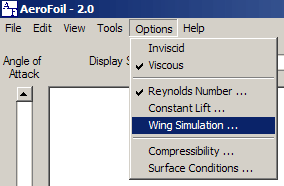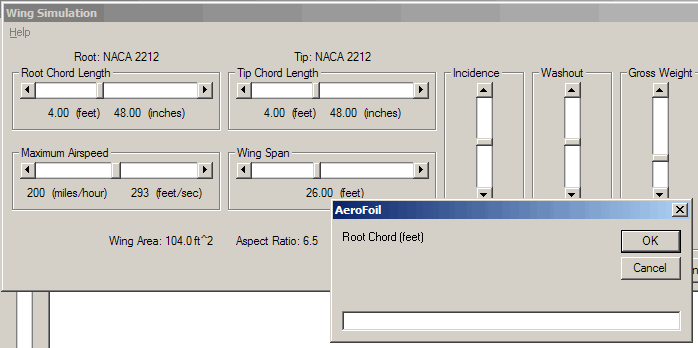## Wing SimulationFor an airplane in stable flight, angle of attack determines the airspeed. Boundary layer development and maximum lift coefficient is highly dependent upon the Reynolds number, which is a function of the airspeed, airfoil length, air density, and air viscosity. In the case of a tapered wing, the Reynolds number and subsequent airfoil characteristics vary over the span of the wing.

From the equation:
Gross Weight = 0.5 * ρ * V2 * Lift Coefficient * Wing Area
the range of airspeed, and hence, Reynolds number may be calculated.

AeroFoil first calculates the minimum angle of attack using the maximum airspeed. The highest angle of attack and minimum airspeed is then found through iteration. The highest angle of attack in this case is assumed to occur when the airfoil at the wing root reaches its maximum coefficient of lift. The range of angle of attack is then sub-divided into equal segments and the airfoil characteristics are found at each.

Incidence represents the angle of attack of the root chord, relative to the fuselage. In aircraft design, the root chord is typically set at an angle of attack that will result in the minimum drag of the airplane at some design condition.

Incidence represents the angle of attack of the root airfoil, relative to the fuselage. A positive value indicates that the root airfoil is at a higher angle of attack than the reference. Washout represents a difference in the angle of attack between the root chord and tip chord. A positive value indicates that the tip is at a lower angle of attack than the root. This is frequently used in aircraft design to ensure that the onset of stall will occur at the wing root and progress towards the tip as the angle of attack is increased. A combination of root chord incidence and tip washout is chosen to establish a level fuselage as some set design condition. In the wing simulation, all tabular results are referenced to the fuselage angle of attack.

Re-arranging the first equation gives:
Cl = Gross Weight / (0.5 * ρ * V2 * Wing Area)

Assuming a semi-elliptic span loading along the wing, then at the wing root:
Cl root = (4 * Cl * Wing Area) / (π * Root Chord * Span)

The mean aerodynamic chord (MAC) represents the average airfoil along the wing's span. In the preliminary calculations of aircraft stability, the wing is assumed to act as if all forces are acting on the MAC. It is found by:
MAC = 2 * [Root + Tip - (Root * Tip)/(Root + Tip)] / 3

If coordinate data for only one airfoil is given, AeroFoil assumes that airfoil is used throughout the wing. If two airfoils are specified, airfoil #1 is used for the root and airfoil #2 is the tip. The MAC is then found by linear interpolation between the two. If three airfoils are specified, airfoil #1 is the root, airfoil #2 is the MAC, and airfoil #3 is the tip.Any numeric value except wing area and aspect ratio may be entered manually by left clicking over the numeric value and typing the desired value in the text box.